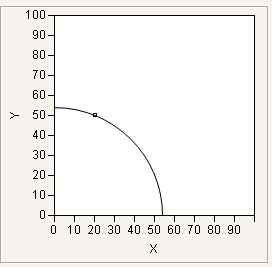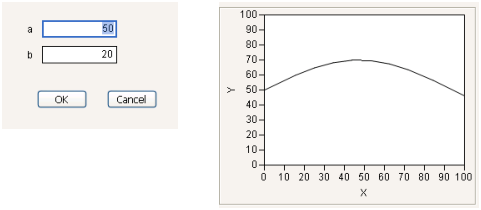Publication date: 11/10/2021

## Scoped Names

Specify where a name is to be resolved by using a scope in the form scope:name where scope indicates how to resolve the name. For example, here:name indicates that the name should be resolved locally. Using the Names Default To Here mode, here:name is equivalent to name. The scope instructs how to look up the name.

The syntax is to use the colon scope operator:

`scope:name`

There are several types of scopes:

Scope can be a resolution rule. For example, here:x means that x should be resolved to a name that is local to the script. Global:x means that x should be resolved to a global name.

Scope can be a namespace reference variable. For example. ref:a means that a should be resolved within the namespace that ref refers to.

Scope can be a data table reference to look up names as column names. For example, dt:height means that height should be resolved as a column in the data table that dt references.

Scope can be the name of a namespace that you created. For example, myNamespace:b where myNamespace is a namespace that you created. "myNamespace":b is equivalent. See Namespaces.

Note: If you create a function, make sure you scope it to avoid conflicts with built-in functions.

#### Examples of Scoping Column Formulas

The following examples demonstrate how to scope columns that contain formulas. In both scripts, x is a global variable, local variable, and column name.

In the first script, the column name x is unscoped. the formula in the second column multiplies the value in column x by 100. In this case, The result is a column with the values 100, 200, and 300.

`::x = 5;`
`New Table( "Test",`
`	New Column( "x", Values( [1, 2, 3] ) ),`
`	New Column( "y", Formula( 100 * x ) ),`
`);`

In the following script, the formula in column y assigns 500 to x and then adds 50 to x. Each cell in the column contains the value 550.

`::x = 5;`
`New Table( "Test",`
`	New Column( "x", Values( [1, 2, 3] ) ),`
`	New Column( "y", Formula( Local( {x = 500}, x + 50 ) ) ),`
`);`

### Predefined Scopes

JMP provides predefined that cannot be removed or replaced. Each of these scopes has specific roles, depending on its associated object.

Table 8.5 Predefined Scopes

Scope

Description

Global

Global names are shared throughout the JMP environment.

Here

Scope of the executing script.

Builtin

JMP built-in functions. For example, Builtin:Sqrt(). These names are shared throughout the JMP environment.

If you over-ride a JSL function with a custom function, you can still access the built-in JSL function by using this scope.

Local

Nearest local scope. Can be nested within the user-defined functions, Local and Parameter.

Local Here

Provides a namespace block inside Names Default to Here(1). Use Local Here() to prevent name collisions when multiple scripts are executed from the same root namespace (for example, when a script executes two button scripts that have the same variables). The argument can be any valid JSL expression.

Local( {Default Local}, ) does not always work due to the lifetime of the local block, but Local Here() is persistent across the call.

Window

Scope of the containing user-defined window. (Rare.)

Platform

Scope of the current platform. (Rare.)

Box

Scope of the containing context box. A context box is nested within a user-defined window. (Rare.)

### Example of Using the Window Scope

This example uses the Window scope to pass information during execution. Explicitly scoping the variables x and y to this window ensures that JMP does not try to scope x and y in other contexts, such as a data table. The variables x and y are created and used solely inside the Window environment. The Window scope is similar to using Local(), but more useful because Local() is limited in the places that it can be used.

`New Window( "Example",`
`	window:gx = 20;`
`	window:gy = 50;`
`	Graph Box(`
`		Frame Size( 200, 200 ),`
`		Handle(`
`			window:gx,`
`			window:gy,`
`			Function( {x, y},`
`				window:gx = x;`
`				window:gy = y;`
`			)`
`		);`
`		Circle( {0, 0}, Sqrt( window:gx * window:gx + window:gy * window:gy ) );`
`	);`
`);`

Figure 8.1 Example of Current Window Namespace### Example of Using the Here Scope

This example uses the Here scope to pass information between windows that are created by the same script. Scoping a variable using Here: is not dependent on turning Names Default To Here() on. The Here: scope is always available.

This script produces two windows and uses two different scopes.

The Launcher window asks the user for two values. Those two values are passed to the Output window, which uses them to graph a function. The Launcher window scopes aBox and bBox to the window: essentially, those two variables (pointers to Number Edit Boxes) exist only in the Launcher window and are not available to the Output window. The values from those two boxes are then copied into variables that are scoped to Here, and so are available to both windows that are produced by this script.

`launchWin = New Window( "Launcher",`
`	<<Modal,`
`	V List Box(`
`		Lineup Box( N Col( 2 ), Spacing( 10 ),`
`			Text Box( "a" ),`
`			window:aBox = Number Edit Box( 50 ),`
`			Text Box( "b" ),`
`			window:bBox = Number Edit Box( 20 ),`
` `
`		),`
`		Lineup Box( N Col( 2 ), Spacing( 20 ),`
`			Button Box( "OK",`
`				// copy values before window goes away`
`				here:a = window:aBox << Get;`
`				here:b = window:bBox << Get;`
`			),`
`			Button Box( "Cancel", Throw( 1 ) )`
`		),`
` `
`	)`
`);`
` `
`New Window( "Output",`
`	Graph Box( Y Function( here:a + here:b * Sin( x / 30 ), x ) )`
`);`

Figure 8.2 Launcher and Output